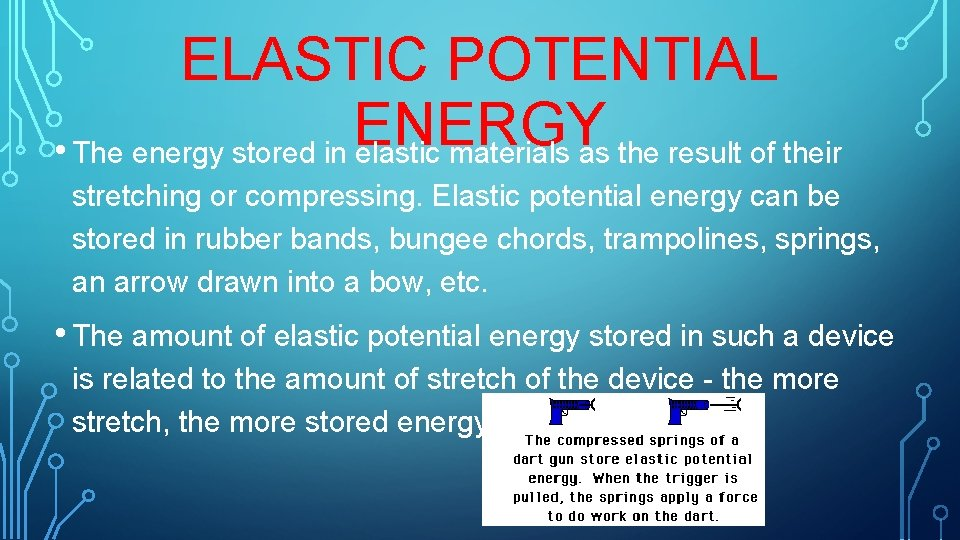# POTENTIAL ENERGY Potential energy is the stored energy

• Slides: 9POTENTIAL ENERGY • Potential energy is the stored energy of position possessed by an object. • For example, the heavy ball of a demolition machine is storing energy when it is held at an2 TYPES OF POTENTIAL ENERGY • Gravitational Energy • Elastic Potential EnergyGRAVITATIONAL POTENTIAL ENERGY • Gravitational Potential Energy is the energy stored in an object as the result of its vertical position or height. • Gravitational Potential Energy is based on mass and height. • More massive objects have greater gravitational potential energy. • The higher that an object is elevated, the greater the gravitational potential energy.GRAVITATIONAL POTENTIAL ENERGY • GPE (PEg) = mgh (mass x gravity x height) • To determine the GPE of an object, a zero height position must first be arbitrarily assigned. Typically, the ground is considered to be a position of zero height.CHECKPOINT Which object has the most potential energy? A 5. 6 kg rock at the top of a mountain 35 meters high, or a 5. 6 kg rock at the top of a mountain 55 meters high?CHECKPOINT • Knowing that the potential energy at the top of the tall platform is 50 J, what is the potential energy at the other positions shown on the stair steps andELASTIC POTENTIAL ENERGY • The energy stored in elastic materials as the result of their stretching or compressing. Elastic potential energy can be stored in rubber bands, bungee chords, trampolines, springs, an arrow drawn into a bow, etc. • The amount of elastic potential energy stored in such a device is related to the amount of stretch of the device - the more stretch, the more stored energy.ELASTIC POTENTIAL ENERGY • Springs are a special instance of a device that can store elastic potential energy due to either compression or stretching. • Such springs are said to follow Hooke's Law. If a spring is not stretched or compressed, then there is no elastic potential energy stored in it. The spring is said to be at its equilibrium position. • PEelastic = ½ kx 2 ( k = spring constant; x = amount of stretch or compression)CLASSWORK • Pg. 172 #’s 1 -2 • Pg. 172 Section Review #’s 1 -3